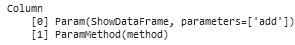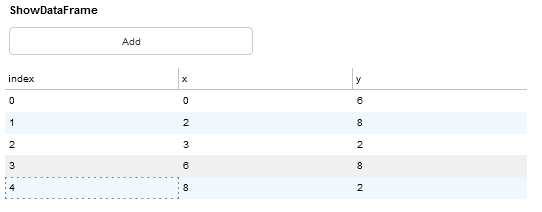# How to access object returned by a paramMethod?

Dear community, I working with `param.Parameterized` classes and often make use of `@pn.depends` decorated methods. In more complex apps, it becomes interesting to access the return object.

E.g. I want to access the returned `pn.widgets.DataFrame` widget to program further interaction with the current `selection`.
However, I hit a dead end with the ´ParamMethod(method)´ object rather than being able to find the actual returned object:Code for my normal way of working (which doesn’t allow to access the returned object):

``````class ShowDataFrame(param.Parameterized):
df = param.DataFrame(default=pd.DataFrame(columns=['x','y']))

def __init__(self,**params):
super().__init__(**params)

self.show_df)

new_point = dict(zip(['x','y'],np.random.randint(0,10,2)))
self.df = self.df.append(new_point,ignore_index=True)

@pn.depends('df')
def show_df(self):
return(pn.widgets.DataFrame(self.df))

t = ShowDataFrame()
t.layout
``````

I’ve been looking into alternatives without using a paramMethod, but direct use of the param.DataFrame doesn’t update the dataframe view.

Closest I’ve come is defining and updating the value of an intermediate `pn.widgets.DataFrame`, which is returned in the panel object. The problem I still face here, besides it feeling like a workaround, is the auto widget `height` is used, which only shows the column names if I start with an empty DataFrame.

``````class ShowDataFrame(param.Parameterized):
df = param.DataFrame(default=pd.DataFrame(columns=['x','y']))

def __init__(self,**params):
super().__init__(**params)
self.df_widget = pn.Param(self.param.df)
self.show_df,
self.update_params_with_selected_row_values)

new_point = dict(zip(['x','y'],np.random.randint(0,10,2)))
self.df = self.df.append(new_point,ignore_index=True)

@pn.depends('df')
def show_df(self):
self.df_widget.value = self.df
return self.df_widget

@pn.depends('df_widget.selection')
def update_params_with_selected_row_values(self):
return self.df_widget.selection

t = ShowDataFrame()
t.layout
``````

Is this approach of predefining a panel object and than updating this object in the watched method, before actually returning this object the only/expected way to do access the returned paramMethod objects?

The height of the pn.widgets.DataFrame widget can be adjusted in the along with the value in the interactive paramMethod. This resolves the issue described above.

``````@pn.depends('df')
def show_df(self):
self.df_widget.value = self.df
self.df_widget.height = int(min(len(self.df)/6,1)*6*self.df_widget.row_height) + self.df_widget.row_height
return self.df_widget
``````

If you’re wanting more control you really should use `watch=True` on your depends decorator:

``````from panel.viewable import Viewer

class ShowDataFrame(Viewer):
df = param.DataFrame(default=pd.DataFrame(columns=['x','y']))

def __init__(self,**params):
super().__init__(**params)
self.df_widget = pn.Param(self.param.df)
self._layout = pn.Column(
self.df_widget,
self.update_params_with_selected_row_values
)

@pn.depends('df', watch=Truee)
def _update_widget(self):
self.df_widget.value = self.df
self.df_widget.height = int(min(len(self.df)/6,1)*6*self.df_widget.row_height) + self.df_widget.row_height

def __panel__(self):
return self._layout

new_point = dict(zip(['x','y'],np.random.randint(0,10,2)))
self.df = self.df.append(new_point,ignore_index=True)

@pn.depends('df_widget.selection')
def update_params_with_selected_row_values(self):
return self.df_widget.selection

t = ShowDataFrame()
t
``````

Performing side-effects in a `ParamMethod` function is really not encouraged, so it’s better to set up the watcher to update the object instead. I’ve also taken the liberty of making your class a so called `Viewer` so that you don’t have to manually access the `.layout` attribute.

The implementation with `watch=True` did come to mind. However, due to the fixed height I didn’t I was seeng the dataframe widget updates, therefore assuming I needed a paramMethod to ensure updates. The direct use of `df_widget = pn.Param(self.param.df)` also releaves the `value` update in `_update_widget` method:
``````@pn.depends('df', watch=True)
I didn’t know about the `Viewer` class. Neat implementation, thanks. Seems like it’s not yet in place in my outdated `panel 0.10.2`.For some reason I’m not eager to update python modules in a project regarding backward compatibilty in my (older) notebooks. I’m getting convinced by all fancy updates, but I need some reassuring that everything’s gonna be alright first…